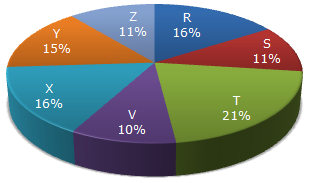# Data Interpretation - Pie Charts

### Exercise :: Pie Charts - Pie Chart 7

Study the following pie-chart and the table and answer the questions based on them.

Proportion of Population of Seven Villages in 1997Village % Population Below Poverty Line X 38 Y 52 Z 42 R 51 S 49 T 46 V 58

1.

If the population of village R in 1997 is 32000, then what will be the population of village Y below poverty line in that year?

 A. 14100 B. 15600 C. 16500 D. 17000

Explanation:

Population of village R = 32000 (given).

Let the population of village Y be y.

 Then, 16 : 15 = 32000 : yy =15 x 32000= 30000. 16Population of village Y below poverty line = 52% of 30000 = 15600.

2.

The ratio of population of village T below poverty line to that of village Z below poverty line in 1997 is:

 A. 11 : 23 B. 13 : 11 C. 23 : 11 D. 11 : 13

Explanation:

Let N be the total population of all the seven villages.

Then, population of village T below poverty line = 46% of (21% of N)

and Population of villages Z below the poverty line = 42% of (11% of N)Required ratio = 46% of (21% of N) = 46 x 21 = 23/11. 42% of (11 % of N) 42 x 11

3.

Find the population of village S if the population of village X below poverty line in 1997 is 12160.

 A. 18500 B. 20500 C. 22000 D. 26000

Explanation:

Let the population of village X be x.

 Then, 38% of x = 12160x = 12160 x 100 = 32000. 38

Now, if s be the population of village S, then

 16 : 11 = 32000 : ss =11 x 3200= 22000. 16

4.

If in 1998, the population of villages Y and V increase by 10% each and the percentage of population below poverty line remains unchanged for all the villages, then find the population of village V below poverty line in 1998, given that the population of village Y in 1997 was 30000.

 A. 11250 B. 12760 C. 13140 D. 13780

Explanation:

Population of village Y in 1997 = 30000 (given).

Let the population of village V in 1997 be v.

 Then, 15 : 10 = 30000 : vv = 30000 x 10 = 20000. 15

Now, population of village V in 1998 = 20000 + (10% of 20000) = 22000.Population of village V below poverty line in 1998 = 58% of 22000 = 12760.